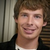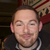+1

# Is there a good way to combine < if> operations with AND or OR?

thillsman 8 years ago updated 8 years ago
I'm working on a Tic Tac Toe app. It's functional, but the code's long. To determine whether the game has been won, I use < if> tags to compare variables like this:

< if lhs="[var:cur1]" operator="e" rhs="[var:cur2]">
< if lhs="[var:cur1]" operator="e" rhs="[var:cur3]">
< alert title="You win!" message="[var:cur1] is the winner!" />
< /if>
< /if>

where the variable are the status of the top three boxes on the tic tac toe board (X or O). Is there a cleaner way to say "if cur1, cur2, and cur 3 are equal, prompt alert"?

Thanks!

PS: Is there a good way to share script on the forums?The best way to share code on the forum is to use html code blocks. Wrap your WIRE examples in < code >< /code > and it should display easily.

As far as your question, you are doing this the way it was designed. Conditional logic must move through a workflow when dealing with multiple objects, so nesting your if statements here is the way to go.

You could do this other ways, but I think you might have the shortest approach here.

Here is another approach that would cause you to do several sets of if statements for each 3 across combination...

``````

<if lhs="[var:cur1]" operator="e" rhs="X">

<if lhs="[var:cur2]" operator="e" rhs="X">

<if lhs="[var:cur3]" operator="e" rhs="X">

</if>

</if>

</if>

<if lhs="[var:cur1]" operator="e" rhs="O">

<if lhs="[var:cur2]" operator="e" rhs="O">

<if lhs="[var:cur3]" operator="e" rhs="O">